# A Tiny Logic Probe TTL / CMOS with Battery Charger

Using an ATtiny1614 microcontroller with LiPo battery, including USB charging circuitry and logic. It is programmable with Arduino IDE!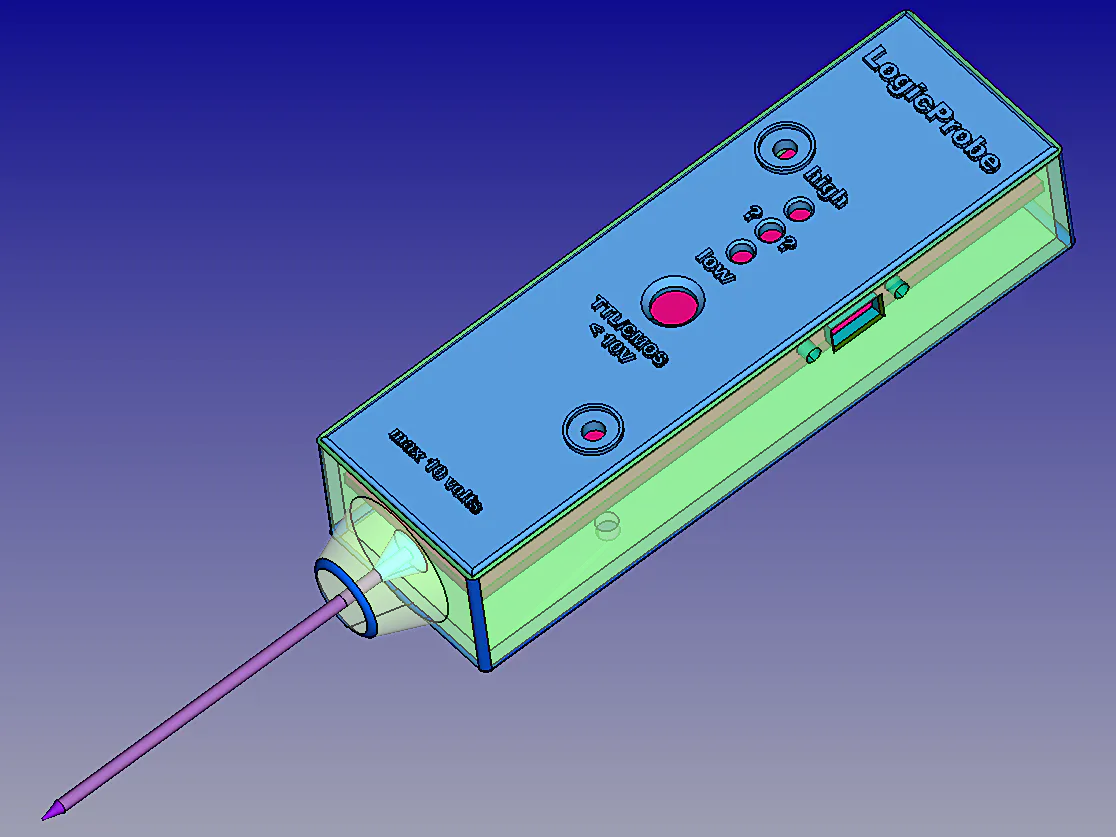## Things used in this project

### Hardware components

 Microchip Technology ATtiny 1614 Processor
×1Arduino Nano R3
×1

## Schematics

### Processor PCB overview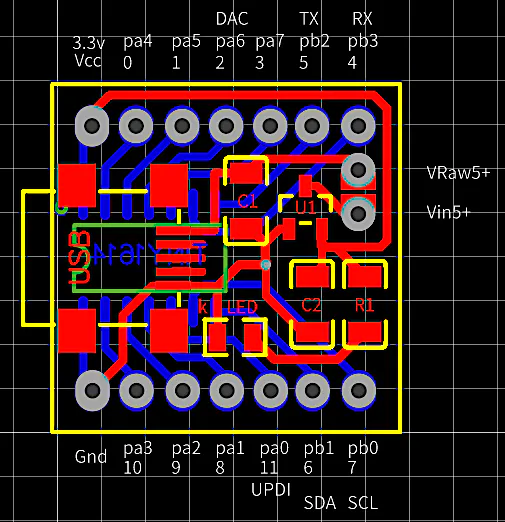### Main PCB overview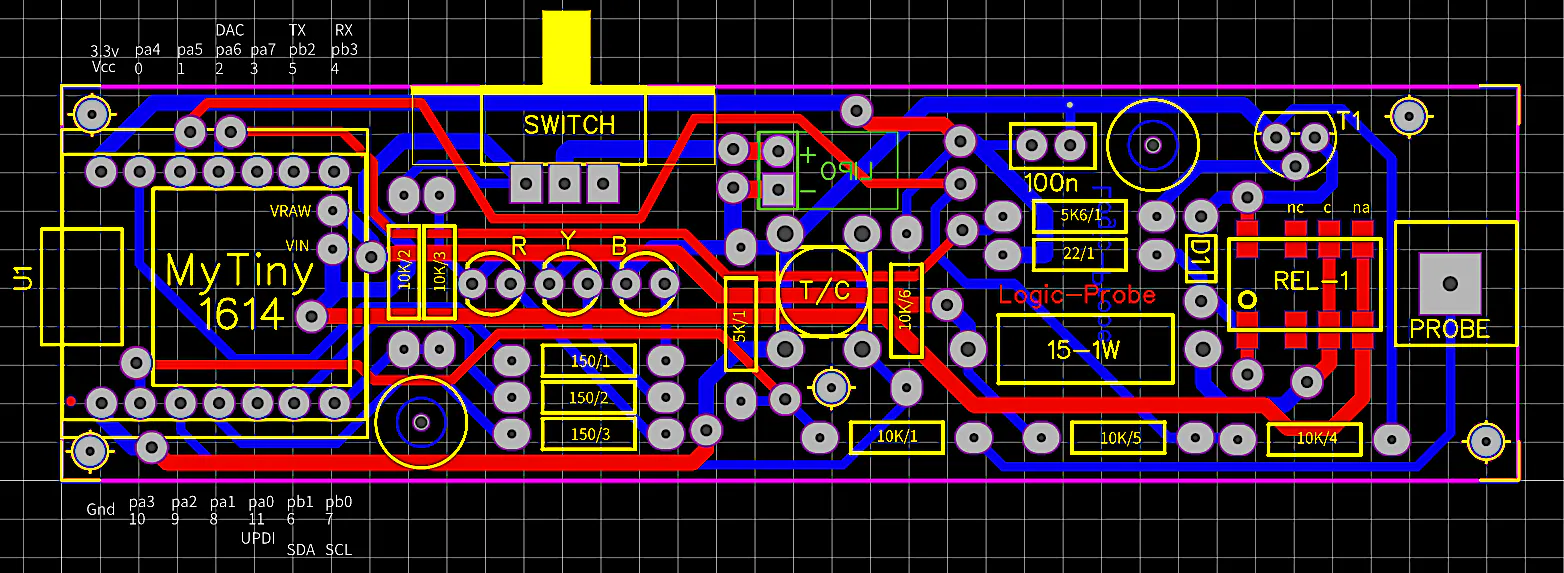### Main PCB bottom face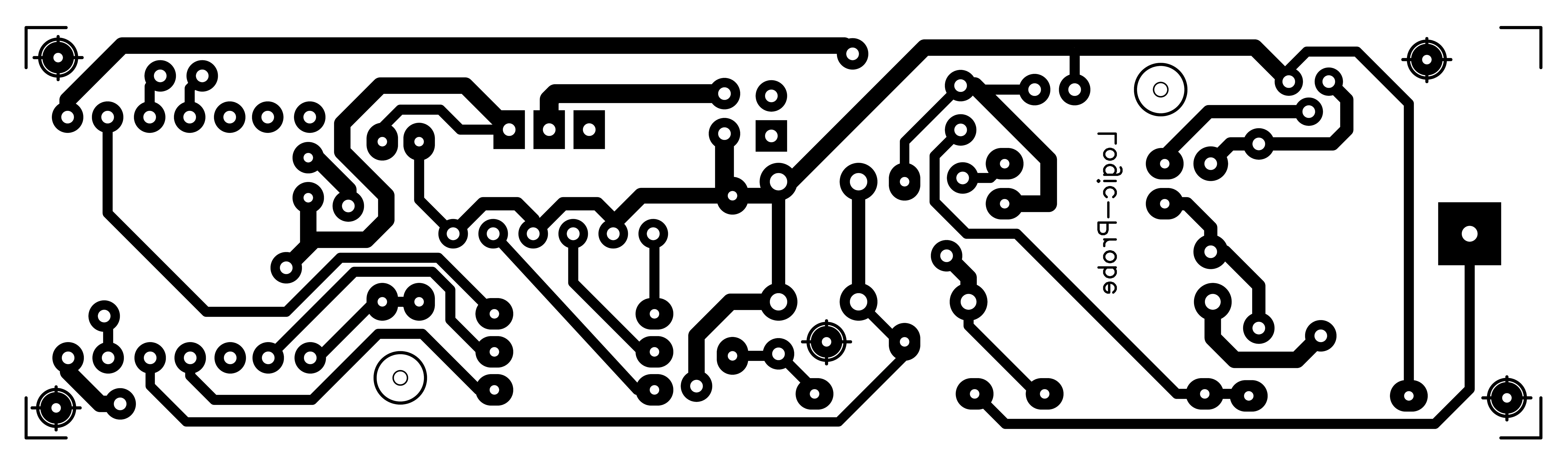### Main PCB top face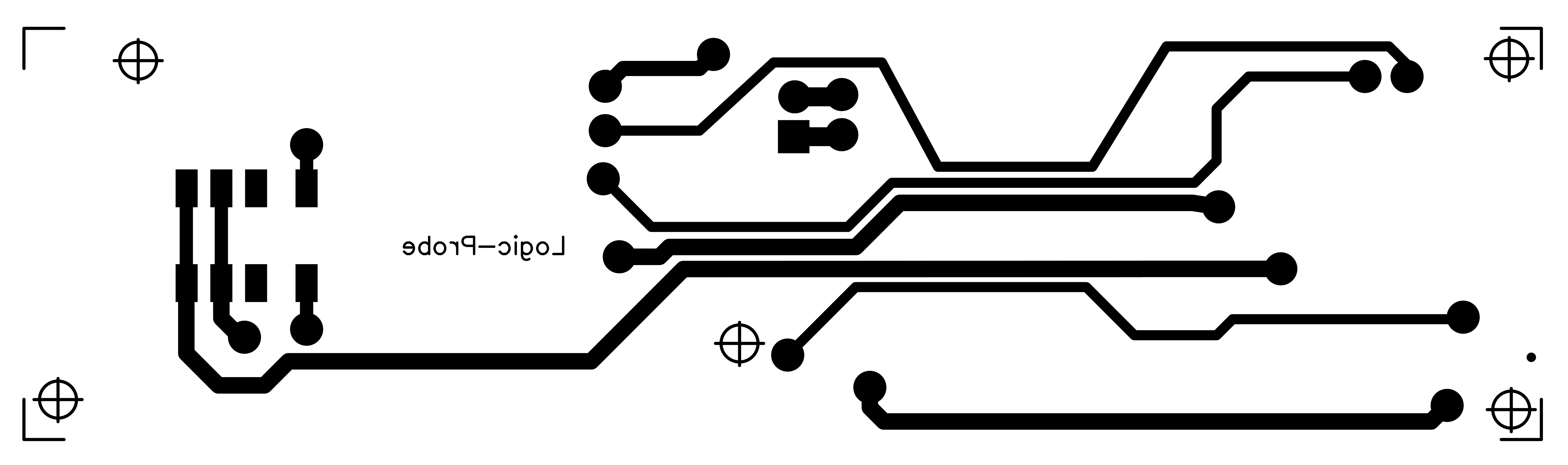### Main PCB components face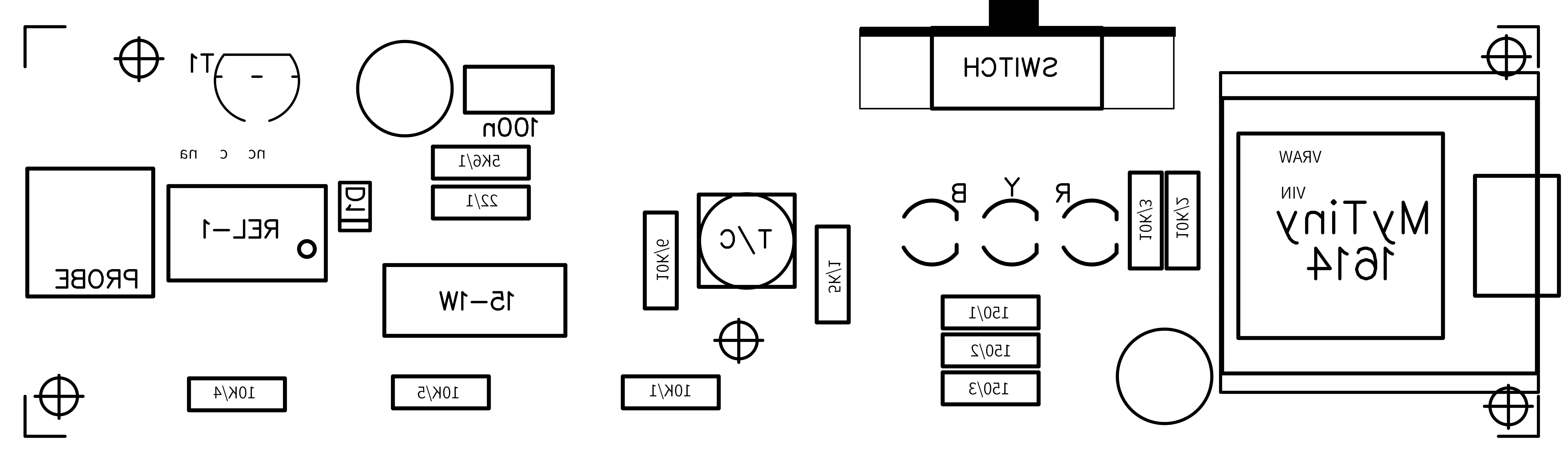### Processor PCB bottom face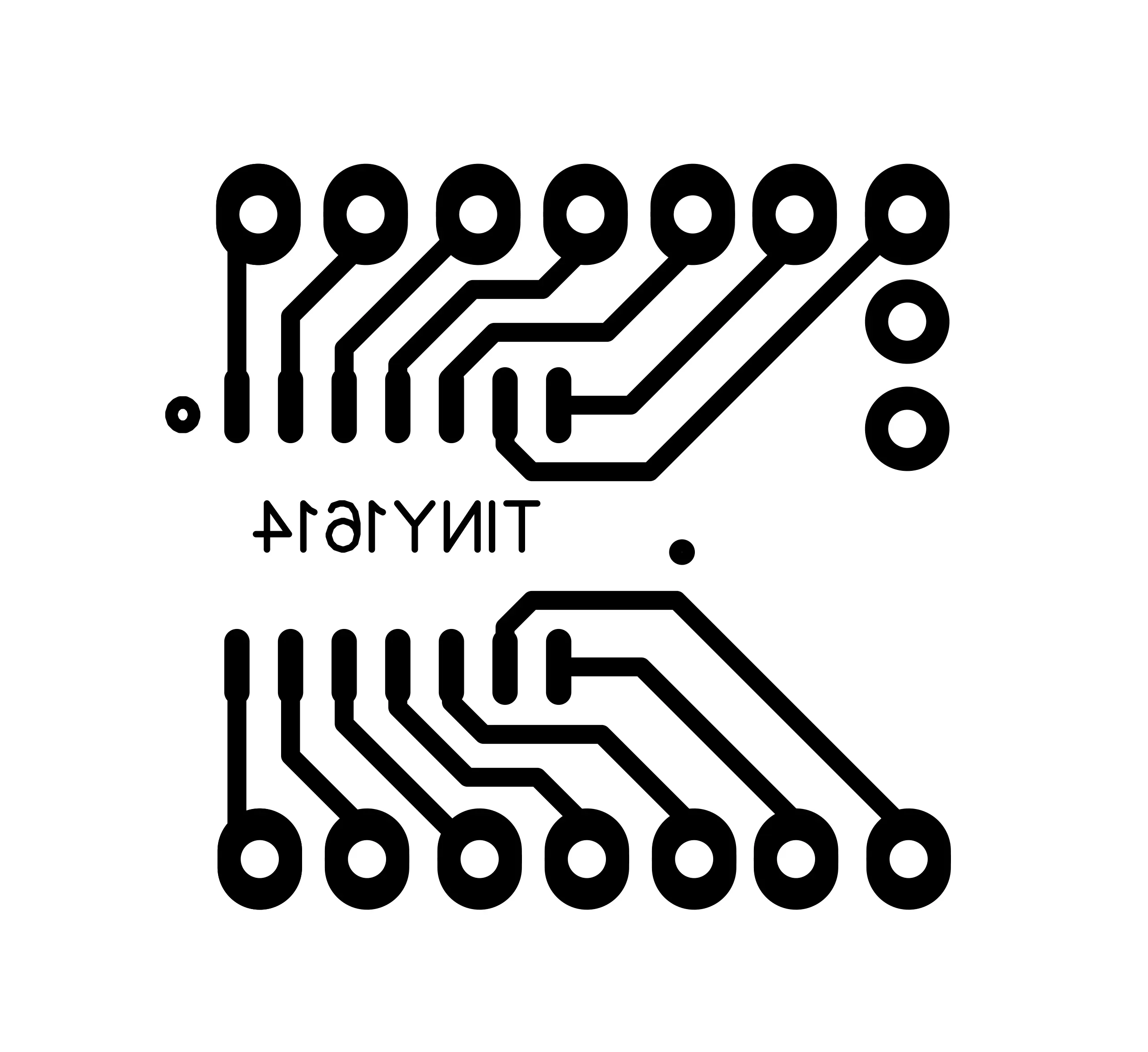### Processor PCB top face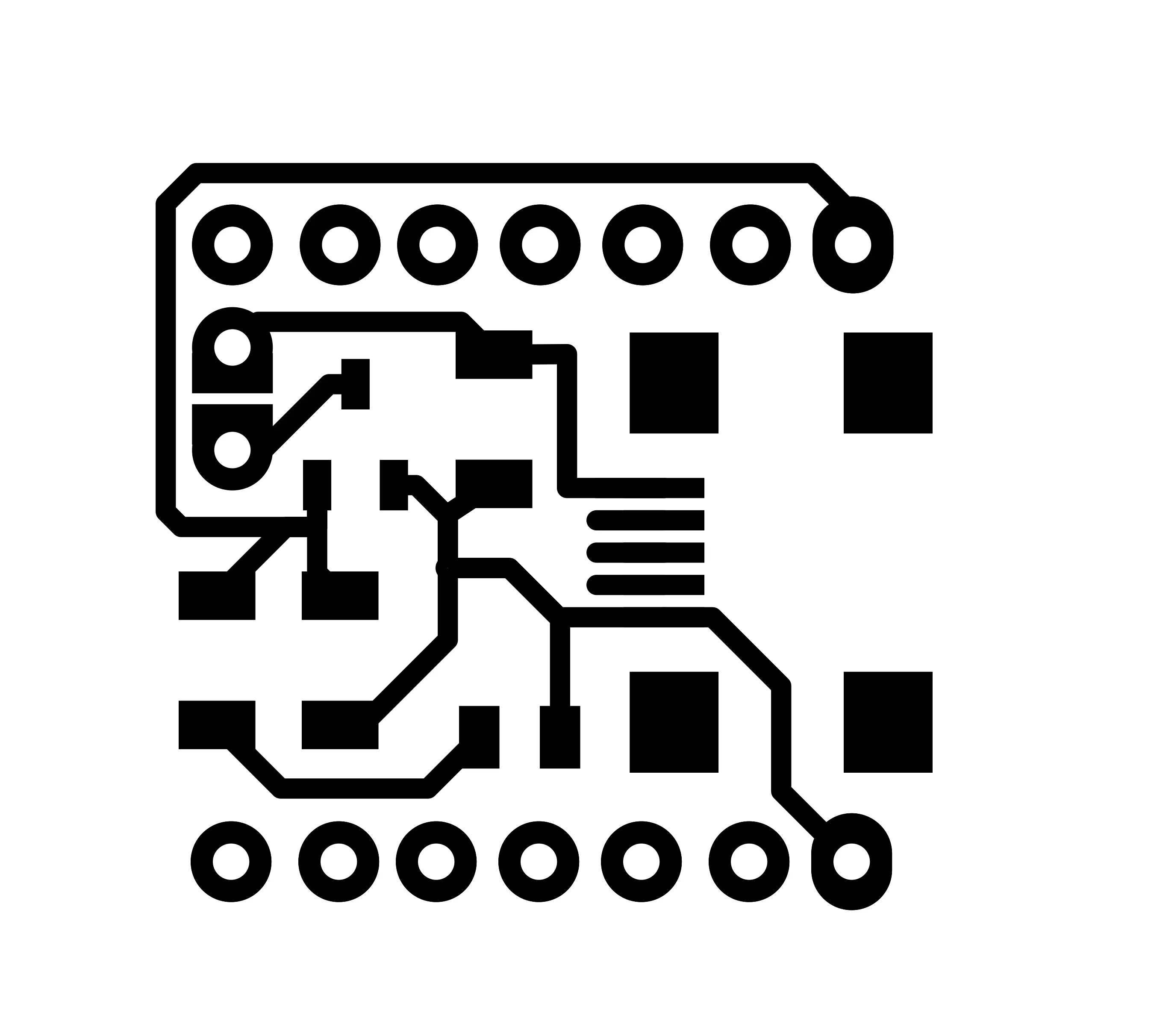### Processor PCB components face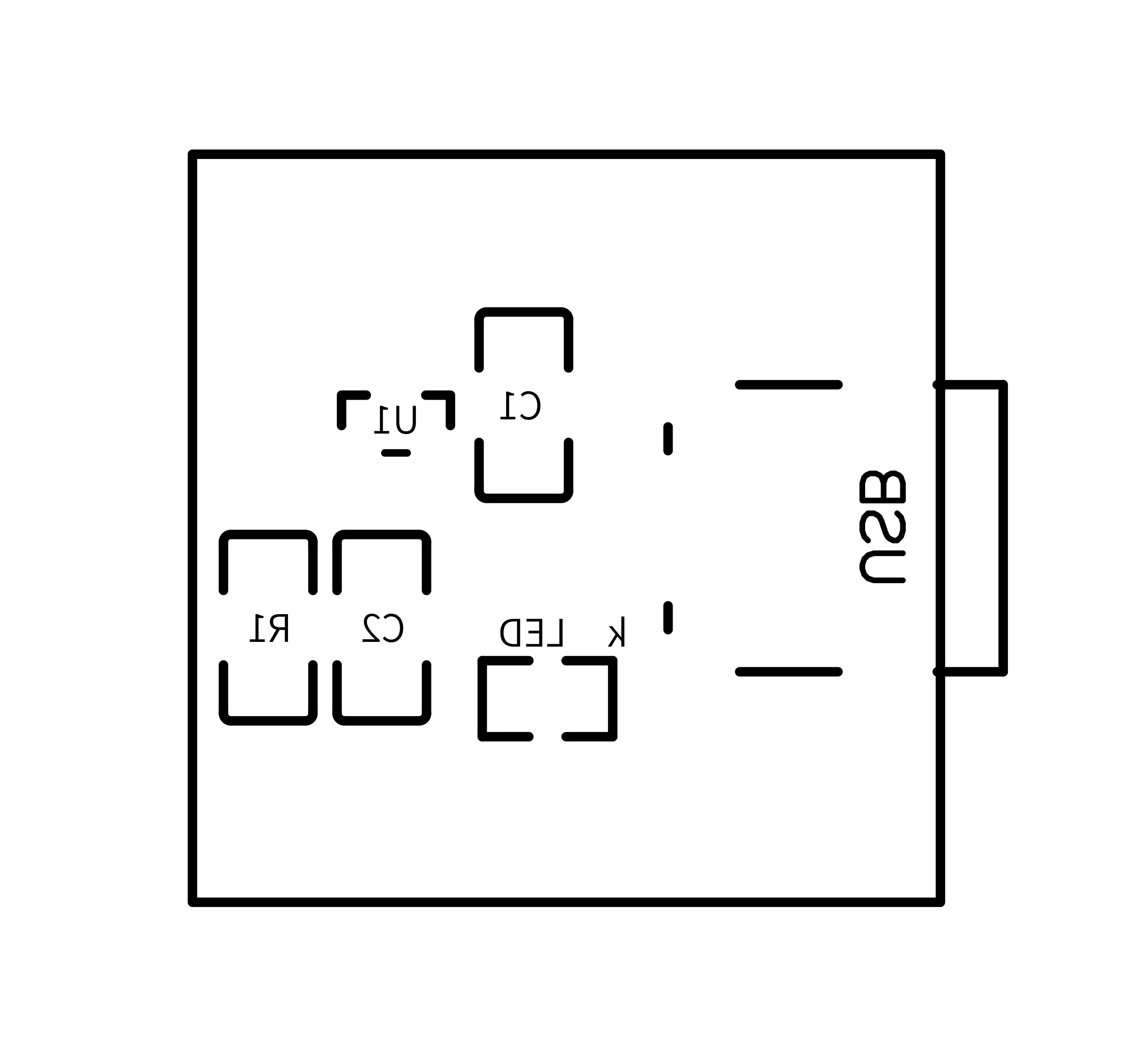## Code

### LogicProbe code

Arduino
```/*
This sketch acts as a logic level tester, by Marco Zonca, 10/2020
ATTINY 1614 as MPU, a button to switch TTL/CMOS (max 10v), 3 x LEDs and a few resistors, etc.;

// ----------------------------------------------------------------------------------------------
//  TTL levels : undefined=yellow >0.8v <= 2.0v  low=blue <= 0.8v  high=red > 2.0v
//  CMOS levels: undefined=yellow >1.5v <= 3.5v  low=blue <= 1.5v  high=red > 3.5v
// ----------------------------------------------------------------------------------------------

As a further step I added a small 300mA/h LiPo battery, code and circuitry to provide charging
functionality: SMD micro relay, NPN BC337, 1N4148 diod and a few more resistors; for
the two couples of 10k resistors as voltage dividers try to select them with similar values as possibile
or you have to apply some +- % of arbitrary correction to the voltage calculation (i.e. VProbe=(n1...))
for every one of them; it is another voltage divider, on probe input, 10k and 5k ohm values.

Charging values:
-----------------s
CBatt = 0.3 (battery power in A/h)
VResis = 15.5 (resistor value in series between PowerSource and Battery, in ohm
please measure the exact resistor value and put it here and below there)
ICirc = 0.05 (estimated maximum current consumption of the circuit without battery charging)

VPower = 5.0 (charging PowerSource voltage from USB) not a constant, it will change in reading
VMinPower = 4.6 (minimum voltage from USB to charge battery)
VBmin = 3.2 (absolute minimum voltage)
VBWmin = 3.4 (working minimum voltage)
VMax = 4.2 (absolute maximum voltage)
VBchgd = 4.0 (battery voltage, or more, to consider it already charged at power-on)
ETFact = 1.5 (estimated time of charging factor)
OvTiFact = 1.2 (overtime of charging factor, in addition to ETFact)
VInitBatt = initial battery voltage, after power-on of just after charged or at start of charging
VBatt = actual battery voltage
IBatt = (( VPower - VBatt ) / VResis) - ICirc (charging current; 0.066A at 0%, 0.034A at 50%, 0.002A at 100%)
IBattAvg = (( VPower - ((VMax+VBmin)/2) ) / VResis) - ICirc = 0.034A (average charging current)
SoC = 100 - (( VMax - VBatt ) * 100) (state of charge in %)
TFull = ( CBatt / IBattAvg ) * 60 = 529 minutes (time of charging at average current and battery at VBmin)
TMaxChg = TFull * ( VMax - VInitBatt ) * ETFact (estimated maximum time of charging considering initial voltage state, in minutes)
TChg = ( TFull * (VMax - VBatt)) * ETFact  (restimated remaining time of charging in minutes)
ChTimeStart = time elapsed as minutes from power-up
ChTimeEnd = time end of charging as minute from power-up
ChTimeNow = time as minutes from power-up
*/

// #include <SoftwareSerial.h>  // can be uncommented for isDEBUG=true and Serial Monitor

// ports in/out
const int ledBluePin = 0;  // pa4, on=LOW lovel (logic 0)
const int ledYellowPin = 6;  // pb1, on=UNDEFINED level in between 0 and 1
const int ledRedPin = 8;  // pa1, on=HIGH level (logic 1)
const int RelayPin = 2;  // pa6, set on (close) or off (open) the relay, due the contact is normally open
const int ProbePin = 10;  // pa3, analog input, logic level will be tested here
const int ButtonPin = 9;  // pa2, open/released=TTL closed/pressed=CMOS
const int VBattPin = 7;  // pb0, analog input, battery voltage is tested here
const int VRawPin = 1;  // pa5, analog input, USB power source voltage is tested here

// Charging constant Values, see notes above
const float CBatt = 0.300;  // put the battery power A/h
const float VResis = 15.5;  // put the exact resistor value
const float ICirc = 0.05;  // put the maximum circuit consumption
const float VMinPower = 4.6;
const float VBmin = 3.2;
const float VBWmin = 3.4;
const float VMax = 4.2;
const float VBchgd = 4.0;
const float ETFact = 1.5;
const float OvTiFact = 1.2;
float VPower = 5.0;  // it will change with read value
float IBattAvg = ((VPower - ((VMax+VBmin)/2) ) / VResis) - ICirc;
float TFull = ( CBatt / IBattAvg ) * 60;

const boolean IsDEBUG = false;  // set this to 'true' for Serial Monitor

const int CLOSE = HIGH;
const int OPEN = LOW;
const float MillisCheckCharge = 4000;
const float MillisDebug = 10000;

// Charging variable Values, see notes above
float VInitBatt = 0;
float VBatt = 0;
float IBatt = 0;
float SoC = 0;
float TMaxChg = 0;
float TChg = 0;
float VRaw = 0;
double ChTimeStart = 0;
double ChTimeEnd = 0;
double ChTimeNow = 0;
bool IsOverTime = false;
bool IsCharging = false;

float prevMillisCheckCharge = 0;
float prevMillisDebug = 0;
bool isCMOS=false;
float n=0;
float n1=0;
float VProbe=0;
byte Status=0;  // 0=0 1=1 2=undefined
byte prevStatus=9;  // 0=0 1=1 2=undefined (previous) [value=9 is just for first loop run]

void setup() {
if (IsDEBUG) Serial.begin(9600);
pinMode(ledBluePin, OUTPUT);
pinMode(ledYellowPin, OUTPUT);
pinMode(ledRedPin, OUTPUT);
pinMode(RelayPin, OUTPUT);
pinMode(ButtonPin, INPUT);
pinMode(ProbePin, INPUT);
pinMode(VBattPin, INPUT);
pinMode(VRawPin, INPUT);
digitalWrite(RelayPin,OPEN);  // disconnects power from battery
digitalWrite(ledBluePin,HIGH);  // LEDs test show
delay(150);
digitalWrite(ledBluePin,LOW);
digitalWrite(ledYellowPin,HIGH);
delay(150);
digitalWrite(ledYellowPin,LOW);
digitalWrite(ledRedPin,HIGH);
delay(150);
digitalWrite(ledRedPin,LOW);
}  // setup()

void loop() {
display();
if ((prevMillisCheckCharge+MillisCheckCharge) < millis()) checkCharge();
if (((prevMillisDebug+MillisDebug) < millis()) && (IsDEBUG)) printDebugging();
}  // loop()

void display() {
digitalWrite(ledBluePin,LOW);
digitalWrite(ledYellowPin,LOW);
digitalWrite(ledRedPin,LOW);
if (VPower > 0) {  // ------------------------- with USB power
if (IsCharging==true) {
if (IsOverTime==false) {
if (SoC > 20 && SoC <=40) blink_red_yellow();
if (SoC > 40 && SoC <=60) blink_yellow();
if (SoC > 60 && SoC <=80) blink_yellow_blue();
if (SoC > 80 && SoC <=90) blink_blue();
} else {
}
} else {
}
} else {
switch (Status) {  // ----------------------- with battery, normal probing operation
case 0:
digitalWrite(ledBluePin, HIGH);
break;
case 1:
digitalWrite(ledRedPin, HIGH);
break;
case 2:
digitalWrite(ledYellowPin, HIGH);
break;
}
prevStatus=Status;
}
}  // display()

if (n > 0) {
n1=(((6.60 * n) / 1023.00));  // 6.60 = 3.30 x 2 (voltage divider /2 in input)
VRaw=(n1 + ((n1 * 0.0) /100));  // arbitrary correction (not active, +-0.0%)
} else {
VRaw=0;
}
VPower=VRaw;
if (n > 0) {
n1=(((6.60 * n) / 1023.00));  // 6.60 = 3.30 x 2 (voltage divider /2 in input)
VBatt=(n1 + ((n1 * 0.0) /100));  // arbitrary correction (not active, +-0.0%)
} else {
VBatt=0;
}
if (VInitBatt==0) VInitBatt=VBatt;  // initial battery voltage, at power-on

void checkCharge() {
if ((IsCharging == false) && (VPower >= VMinPower) && (VBatt < VBchgd)) {
ChTimeStart=int((millis()/1000)/60);  // start charging, elapsed time as minutes from power-up
digitalWrite(RelayPin,CLOSE);  // connets power to battery
VInitBatt=VBatt;  // initial charging battery voltage
IsOverTime=false;
IsCharging=true;
}
if ((IsCharging == true) && ((VPower < VMinPower) || (VBatt >= VMax))) {
ChTimeEnd=int((millis()/1000)/60);  // end charging, elapsed time as minutes from power-up
digitalWrite(RelayPin,OPEN);  // disconnects power from battery
VInitBatt=VBatt;  // battery voltage, just after charging is finished
IsCharging=false;
}
IBatt = ((VPower - VBatt) / VResis) - ICirc; // charging current; 0.066A at 0%, 0.034A at 50%, 0.002A at 100%
IBattAvg = ((VPower - ((VMax+VBmin)/2) ) / VResis) - ICirc; // 0.034A average charging current
SoC = 100 - ((VMax - VBatt) * 100); // state of charge in %
TFull = (CBatt / IBattAvg) * 60;  // 529 minutes, time of charging at average current and battery at VBmin
TMaxChg = TFull * (VMax - VInitBatt) * ETFact * OvTiFact; // estimated maximum time of charging considering initial voltage state, in minutes
TChg = (TFull * (VMax - VBatt)) * ETFact;  //  estimated remaining time of charging in minutes
ChTimeNow=int((millis()/1000)/60);  // now time as minutes from power-up
if ((ChTimeNow-ChTimeStart) > TMaxChg) IsOverTime=true;
prevMillisCheckCharge=millis();
}  // checkCharge()

isCMOS=true;
} else {
isCMOS=false;
}
if (n > 0) {
n1=(((9.90 * n) / 1023.00));  // 9.90 = 3.30 x 3 (voltage divider /3 in input)
VProbe=(n1 + ((n1 * 0.0) /100));  // arbitrary correction (not active, +-0.0%)
} else {
VProbe=0;
}
if (isCMOS==false) {  // TTL case
if (VProbe <= 0.8) {
Status=0;
}
if (VProbe > 0.8 && VProbe <= 2.0) {
Status=2;
}
if (VProbe > 2.0) {
Status=1;
}
}
if (isCMOS==true) {  // CMOS case
if (VProbe <= 1.5) {
Status=0;
}
if (VProbe > 1.5 && VProbe <= 3.5) {
Status=2;
}
if (VProbe > 3.5) {
Status=1;
}
}

delay(500);
digitalWrite(ledRedPin, HIGH);
}
delay(500);
digitalWrite(ledBluePin, HIGH);
delay(500);
}
delay(500);
digitalWrite(ledYellowPin, HIGH);
delay(500);
}
delay(500);
digitalWrite(ledRedPin, HIGH);
delay(500);
digitalWrite(ledRedPin, LOW);
digitalWrite(ledYellowPin, HIGH);
delay(500);
}
delay(500);
digitalWrite(ledYellowPin, HIGH);
delay(500);
digitalWrite(ledYellowPin, LOW);
digitalWrite(ledBluePin, HIGH);
delay(500);
}
delay(1000);
digitalWrite(ledBluePin, HIGH);
delay(2000);
}
delay(100);
digitalWrite(ledYellowPin, HIGH);
delay(100);
}
delay(100);
digitalWrite(ledBluePin, HIGH);
delay(100);
}
void printDebugging() {
Serial.println("----------------------------------------------");
if (IsCharging) {
Serial.print("IsCharging=");
Serial.println(IsCharging);
Serial.print("VRaw (VPower)=");
Serial.println(VRaw);
Serial.print("VInitBatt=");
Serial.println(VInitBatt);
Serial.print("VBatt=");
Serial.println(VBatt);
Serial.print("IBatt=");
Serial.println(IBatt);
Serial.print("IBattAvg=");
Serial.println(IBattAvg);
Serial.print("SoC%=");
Serial.println(SoC);
Serial.print("TFull=");
Serial.println(TFull);
Serial.print("TMaxChg max minutes, more is OverTime=");
Serial.println(TMaxChg);
Serial.print("TChg remaining minutes=");
Serial.println(TChg);
Serial.print("ChTimeStart=");
Serial.println(ChTimeStart);
Serial.print("TimeNow=");
Serial.println(ChTimeNow);
Serial.print("IsOverTime=");
Serial.println(IsOverTime);
Serial.print("VProbe=");
Serial.println(VProbe);
} else {
Serial.print("IsCharging=");
Serial.println(IsCharging);
Serial.print("VRaw (VPower)=");
Serial.println(VRaw);
Serial.print("VInitBatt=");
Serial.println(VInitBatt);
Serial.print("VBatt=");
Serial.println(VBatt);
Serial.print("SoC%=");
Serial.println(SoC);
Serial.print("ChTimeStart=");
Serial.println(ChTimeStart);
Serial.print("ChTimeEnd=");
Serial.println(ChTimeEnd);
Serial.print("TimeNow=");
Serial.println(ChTimeNow);
Serial.print("Millis()= ");
Serial.println(millis());
Serial.print("VProbe=");
Serial.println(VProbe);
}
prevMillisDebug=millis();
}  // printDebugging()
```

## Credits

### marcozonca

4 projects • 22 followers